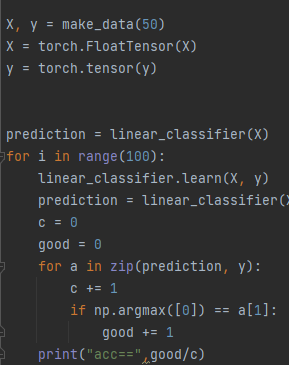# My very simpe net don't learn

I made a simple net and very simple data. (just want learn pytorch) .

data
def make_data(nr_of_data):
x_list = []
y_list = []
for i in range(nr_of_data):
x = [random.randint(0, 1), random.randint(0, 1)]
y = x
x_list.append(x)
y_list.append(y)
return x_list, y_list

model
class LinearClassifier(nn.Module):
def init(self, lr, n_classes, input_dims):
super(LinearClassifier, self).init()
self.linear_1 = nn.Linear(input_dims, 128)
self.linear_2 = nn.Linear(128, 256)
self.linear_3 = nn.Linear(256, n_classes)
self.loss = nn.CrossEntropyLoss()
self.device = T.device(“cuda:0” if T.cuda.is_available() else “cpu”)
self.to(self.device)

``````def forward(self, data):
result = F.relu(self.linear_1(data))
result = F.relu(self.linear_2(result))
result = self.linear_3(result)
return result

def learn(self, data, labels):
data = T.tensor(data).to(self.device)
labels = T.tensor(labels).to(self.device)
predictions = self(data)
cost = self.loss(predictions, labels)
cost.backward()
self.optimizer.step()
``````

and model just can’t learn that simple data .
why ?

I will highly recommend you go with this tutorial instead.

How are you observing that your net isn’t learning? Are the parameters not changing, loss isn’t decreasing, or via some other metric?

I’d consider checking if the model can overfit a small dataset (e.g., a single batch) with a very small learning rate as a sanity check.

i made very simple dada (you can see code to create it )
and give 100 epoch for learn and get 0 accurace increase
(loss decrese )

How are you measuring the accuracy and is the test data the same as the training data? (I wouldn’t expect a model to be able to generalize from one random dataset to another).

X, y = make_data(50)
X = torch.FloatTensor(X)
y = torch.tensor(y)

for i in range(100):
linear_classifier.learn(X, y)
prediction = linear_classifier(X)
c = 0
good = 0
for a in zip(prediction, y):
c += 1
if np.argmax() == a:
good += 1
print(“acc==”,good/c)

And i use only train data (i just want learny torch “API” so i want just overfit very simple data but i can’t XD )sorry i can’t edit text . My first post (:

Ach and data is not random . The target is just a first argument of data y=x

I believe there is just a small typo in the code, please try this loop instead:

``````for i in range(100):
linear_classifier.learn(X, y)
prediction = linear_classifier(X)
c = 0
good = 0
for a in zip(prediction, y):
c += 1
if np.argmax(a) == a:
good += 1
print("acc==",good/c)
``````

(argmax of just 0 was called in the original)
Note that you can also use `torch.argmax` with `axis=1` instead of numpy as well.